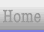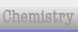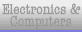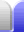# Sequences: Homework Matchsticks

### Marking scheme

Marking points will be allocated thus:

• 6 points presentation for:
• large table
• colour
• photographs of matchstick diagrams, not just rough drawings.
• 4 points completeness for:
• a reasonable number of rows in the table, like 5 or 6
• a row in the table for the generic case
• a row in the table for a high matchstick count
• a check column
• 10 points accuracy and working for:
• clearly showing working and calculations
• correct formula
• checking formula actually works for first few values
• application of formula for number(s) outside the first few that are easily countable

### Table

 n diagram matchstick count formula check 1horizontal = 2 vertical = 2 total = 4 2n(n+1) 2 * 1 * 2 = 4 √ 2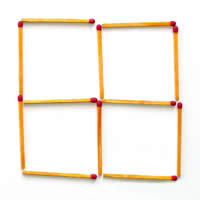horizontal = 2 * 3 = 6 (2 matches in each of 3 rows) vertical = 2 * 3 = 6 (2 matches in each of 3 columns) total = 12 2n(n+1) 2 * 2 * 3 = 12 √ 3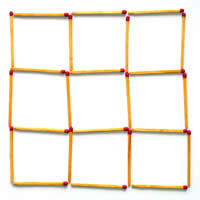horizontal = 3 * 4 = 12 (3 matches in each of 4 rows) vertical = 3 * 4 = 12 (3 matches in each of 4 columns) total = 24 2n(n+1) 2 * 3 * 4 = 24 √ 4horizontal = 4 * 5 = 20 (4 matches in 5 rows) vertical = 4 * 5 = 20 (4 matches in 5 columns) total = 40 2n(n+1) 2 * 4 * 5 = 40 √ 5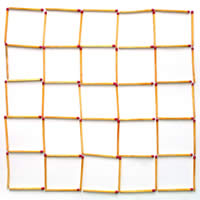horizontal = 5 * 6 = 30 (5 matches in each of 6 rows) vertical = 5 * 6 = 30 (5 matches in each of 6 columns) total = 60 2n(n+1) 2 * 5 * 6 = 60 √ 6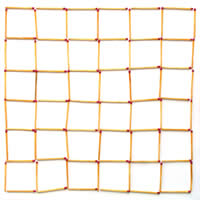horizontal = 6 * 7 (6 matches in each of 7 rows) vertical = 6 * 7 (6 matches in each of 7 columns) total = 84 2n(n+1) 2 * 6 * 7 = 84 √ 100(To count the matches, click for a larger version.) lots 2n(n+1) 2 * 100 * 101 = 202 * 100 = 20,200

There are several ways the formula could be written. The first is probably the easiest to compute in one's head.

f(n) = 2n(n + 1)

f(n) = 2(n2 + n)

f(n) = 2n2 + 2n

Another way to work out the number of matches used would be to count the number of squares, then subtract the number of matches from those squares that would be doubled up.

4 matches in each of n * n squares
∴ matches in squares = 4n2

n matches doubled up in each of (n-1) rows = n(n-1)
n matches doubled up in each of (n-1) columns = n(n-1)
∴ matches doubled up = 2n(n-1)

∴ total matches = 4n2 - 2n(n-1)
= 4n2 - (2n2 - 2n)
= 4n2 - 2n2 + 2n
= 2n2 + 2n
= 2n(n+1)

Not surprisingly, it comes out with the same formula.Home Astronomy Chemistry Electronics & Computers Mathematics Physics Field Trips Turn on javascript for email link